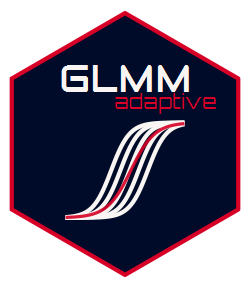Fits generalized linear mixed models for a single grouping factor under maximum likelihood approximating the integrals over the random effects with an adaptive Gaussian quadrature rule; Jose C. Pinheiro and Douglas M. Bates (1995) .Description

GLMMadaptive fits mixed effects models for grouped/clustered outcome variables for which the integral over the random effects in the definition of the marginal likelihood cannot be solved analytically. The package approximates these integrals using the adaptive Gauss-Hermite quadrature rule.

Multiple random effects terms can be included for the grouping factor (e.g., random intercepts, random linear slopes, random quadratic slopes), but currently only a single grouping factor is allowed.

Basic Features

• The package contains a single model-fitting function named mixed_model() with four required arguments, fixed a formula for the fixed effects, random a formula for the random effects, family a family object specifying the type of response variable, and data a data frame containing the variables in the previously mentioned formulas.

• Methods for standard generics are provided, i.e., coef(), fixef(), ranef(), vcov(), logLik(), summary(), anova(), confint(), fitted(), residuals(), predict(), and simulate().

• Negative binomial mixed models can be fitted using the negative.binomial() family object.

• Zero-inflated Poisson and negative binomial models using the zi.poisson() and zi.negative.binomial() family objects.

• Hurdle Poisson and negative binomial models using the hurdle.poisson() and hurdle.negative.binomial() family objects.

• Two-part/hurdle mixed models for semi-continuous normal data using the hurdle.lognormal() family objects.

• Beta and hurdle Beta mixed effects models using beta.fam() and hurdle.beta.fam() family objects.

• Users may also specify their own log-density function for the repeated measurements response variable, and the internal algorithms will take care of the optimization.

• Calculates the marginalized coefficients using the idea of Hedeker et al. (2017) using function marginal_coefs().

• Predictions with confidence interval for constructing effects plots are provided by function effectPlotData().

Basic Use

Let y denote a grouped/clustered outcome, g denote the grouping factor, and x1 and x2 covariates. A mixed effects model with y as outcome, x1 and x2 as fixed effects, and random intercepts is fitted with the code:

In the data argument we provide the data frame DF, which contains the aforementioned variables. In the family argument we specify the distribution of the grouped/clustered outcome conditional on the random effects. To include in the random-effects part intercepts and x1, we update the call to mixed_model() as

Installation

The development version of the package can be installed from GitHub using the devtools package:

and with vignettes

Hex-sticker courtesy of Greg Papageorgiou @gr_papageorgiou.

News

General

• Added support for the DHARMa package.

• The new vignette Goodness of Fit for MixMod Objects describes how to check the fit of mixed models fitted by GLMMadaptive.

• Added support for the effects package.

• There is a new section in the vignette Methods for MixMod Objects illustrating the use of the effects package.

• Function marginal_coefs() has a faster implementation. Compared to the previous implementation the results will be slightly different.

• The optimizer nlminb() can now also be invoked using the new control argument optimizer; default is "optim" corresponding to function optim().

• The new vignette Optimization and Numerical Integration in GLMMadaptive describes how to control the optimization and numerical integration procedures in the package.

General

• The predict() method now works for zero-inflated and hurdle models.

• Hurdle Beta mixed effects models are now available using the hurdle.beta.fam family object.

• The new function scoring_rules() calculates proper scoring rule for subject-specific predictions from mixed models for categorical data.

• Added support for the emmeans package.

General

• Hurdle Poisson and negative binomial models are now implemented using the family objects hurdle.poisson and hurdle.negative.binomial, respectively.

• added S3 methods for the terms(), model.frame() and model.matrix() generics in order to work with the multcomp package.

• A new vignette illustrating multiple comparisons with the multcomp package.

• Methods vcov(), summary(), confint(), anova(), marginal_coefs(), effectPlotData(), predict(), and simulate() have gained the logical argument sandwich to invoke the use of robust/sandwich standard errors in the calculations.

General

• Zero-inflated Poisson and negative binomial models are now implemented using the family objects zi.poisson() and zi.negative.binomial(), respectively. In addition, taking into advantage of the fact that users can specify their own log density functions for the outcome, two-part / hurdle model can also be implemented.

• A new vignette illustrates how the zero-inflated models can be fitted.

• The predict() method is now fully available. It calculates predictions, and standard errors for models returned by mixed_model() at three levels:

• "mean subject": only the fixed effects part corresponding to predictions for the average subject (but not population averaged predictions in case of nonlinear link functions).

• "marginal": predictions using the marginalized coefficients that correspond to population averaged predictions.

• "subject specific": predictions at the subject level. These can be also calculated for subjects not originally in the dataset (i.e., estimates of the random effects are internally obtained).

• The simulate() method is available to simulate data from fitted mixed models. This can be used for instance to perform replication / posterior predictive checks.

General

• First version of the package.

Reference manual

0.8-2 by Dimitris Rizopoulos, 6 months ago

Authors: Dimitris Rizopoulos [aut, cre]

Documentation:   PDF Manual